# General Stochastic Clocks

This is the first post in a series on asset price dynamics that are subject to a stochastic clock. The main idea is to not model the volatility as a stochastic process but instead make the rate at which time progresses randomly. In this post, we outline the basic idea and define the terminology.

Related Posts

Asset Price Model

Letbe a Lévy process and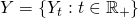be a differentiable finite-variation time-change process on a filtered probability space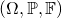. We recall that as a time-change,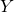has initial value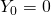and is a right-continuous, strictly increasing process, see e.g. Chapter IV.3 in Protter (2004). We interpret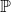to be the physical probability measure and model the stock price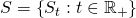as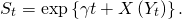Here,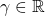is some constant, not to be confused with the real-world drift of the stock price. We will elaborate on this in a later post, when discussing measure changes for stochastic clock models. Since the time-changeis differentiable and of finite-variation, it can be represented as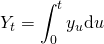for some process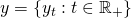. Thus,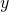is the instantaneous rate of activity process. From the definition ofis follows thathas to be strictly positive. In the following post, we discuss the case wherefollows a non-Gaussian Ornstein-Uhlenbeck process.

The economic interpretation of this setup is thatmodels the rate of information arrival. It captures the effect that asset prices change as new information becomes available. The volatility of these changes is the higher, the more information or the more significant information is released.

Characteristic Function

We are now interested in finding the characteristic functionof the logarithmic asset price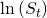. By definition,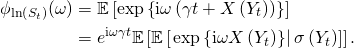Here, we conditioned on the value of the time-change in the second step. Now let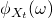be the characteristic function of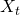. Since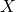is a Lévy process, it is given in terms of its characteristic tripletby the Lévy-Khinchin representation, see e.g. Theorem 3.1 in Cont and Tankov (2004) or Theorems 1.2.14 and 1.3.3 in Applebaum (2004). Furthermore, letting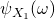be the corresponding characteristic exponent, it takes the formsee e.g. Proposition 3.2 in Cont and Tankov (2004) or Theorem 1.3.3 Applebaum (2004). Thus,Now let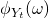be the characteristic function of the time-change, then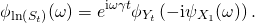In the next post, we will find a general expression for.

References

Applebaum, David (2004) Lévy Processes and Stochastic Calculus: Cambridge University Press

Cont, Rama and Tankov, Peter (2004) Financial Modelling with Jump Processes: Chapman & Hall

Protter, Philip E. (2004) Stochastic Integration and Differential Equations: Springer